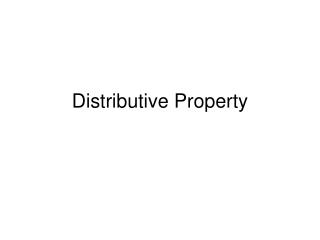DownloadDownload PresentationDistributive Property

# Distributive Property

Télécharger la présentation## Distributive Property

- - - - - - - - - - - - - - - - - - - - - - - - - - - E N D - - - - - - - - - - - - - - - - - - - - - - - - - - -
##### Presentation Transcript

1. Distributive Property

2. Distributive Property • The property that states that multiplying a sum by a number is the same as multiplying each addend in the sum by the number and then adding the products. • 7 x 15 7 x (10 + 5) (7 x 10) + (7 x 5) 70 + 35 105

3. Distributive Property • Decide which number will be the sum • Take that sum apart into easier addends (expanded notation recommended) • Multiply each addend with the factor • Add the partial products together Distributive Property is represented as… a(b + c) = (a x b) + (a x c), a(b + c) = ab + ac for any value of a, b, and c